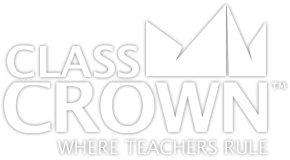Homeroom
Products
Store
Math Worksheets
BlogMath Worksheets

# 2nd Grade Math Worksheets: Pack 1

## Self-checking math worksheets with riddles to keep kids motivated.

Rating: 0/5
(0 Reviews)
2nd
WORKSHEET COUNT
30
FORMAT(S)
PDF
Yes
\$8.99
This comprehensive bundle includes 2nd grade packs 1–2.
\$14.49
\$17.98
Save 19%
The Details
Description
• Do your students ever complete an entire math worksheet, only to find that they have done most of the problems incorrectly and clearly have a misconception?
• ClassCrown’s Riddle-Me-Worksheets help to prevent this problem by introducing a puzzle aspect to math, giving students immediate feedback as to whether or not they are solving problems correctly. If the answer to the riddle isn't correct, the student knows which problems he's made an error on.
Specifics
• Subject:  Addition, Subtraction, Measurement, +more!
• Product Count:  30
• Full Page Count:  56
• File Format(s):  PDF

What's Included?
• Solving addition word problems within 100
• Solving two-step addition word problems
• Solving two-step word problems involving addition and subtraction
• Subtracting with 100
• Solving subtraction word problems within 100
• Adding and subtracting within 20
• Adding and subtracting within 20 (using a symbol to stand in as a number)
• Finding even and odd numbers of shapes
• Creating even equations
• Creating addition equations from arrays
• Creating multiplication equations from arrays
• Measuring in inches
• Comparing two measurements
• Estimating measurements
• Measuring to determine the difference in length
• Solving word problems involving lengths in the same unit
• Adding and subtracting with number lines
• Telling time from analog clocks to the half hour
• Telling time from analog clocks to the quarter hour
• Telling time from analog clocks in 5 minute increments
• Counting money, pennies and dimes
• Counting money, nickels and dimes
• Counting money, dimes, nickels, and pennies
• Counting money, quarters, dimes, nickels, and pennies
• Counting money, dollars and cents
• Counting money word problems
• Measuring and creating line plots
CCSS: 2.OA.A.1, 2.OA.B.2, 2.OA.C.3, 2.OA.C.4, 2.MD.A.1, 2.MD.A.2, 2.MD.A.3, 2.MD.A.4, 2.MD.B.5, 2.MD.B.6, 2.MD.C.7, 2.MD.C.8, 2.MD.C.9, 2.MD.C.10
Reviews
Overall Rating: 0/5
(Showing 0 of 0)
See More Reviews @ TpTSelf Checking

Our math worksheets introduce a puzzle aspect to math, giving students immediate feedback as to whether or not they are solving problems correctly. If the answer to the riddle isn't spelled correctly, the student knows which problems he's made an error on.Fun Puzzle AspectImmediate FeedbackProblem Solving Motivation

Each math worksheet contains a riddle that the student solves by completeing all the problems on the worksheet. This keeps kids motivated to complete each problem so that they can find the answer to the riddle.Common Core Aligned

All our math worksheet packs are designed with Common Core in mind. That way you don’t have to worry about whether your math ciriculum is aligned or not when you incorpoate ClassCrown Riddle-Me-Worksheets in your lesson plans.High Quality Design

Each page of our math worksheets has been produced in high resolution at 144 dpi and designed in full, vibrant color for maximum quality. They look stunning whether you are printing in color or black and white.High Resolution
(144 dpi)Stunning Color & Clarity